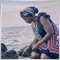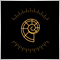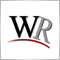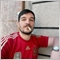# How to calculate correct LOT size for NAS100 - page 348

Keith Watford:

I should double check that if I were you.

I am not aware of any broker that has 1 contract to be any thing other than the unit cost.

If it is, then my calculations will not be any good.

Your calculations is still good. I just adjust for the contract size.

I asked for help with a real live example for me to understand the calculations. Not for a sum to copy and paste.

Thanks again with that.9

With my type of account the tick value is \$1 per tick and this value change depending on type of account you open with your broker. so with 1 factor tick value to get the amount of my stop-loss in \$ I just have to divide the amount I am will to loss on a trade in \$ by the number of pips for my stop-loss For example Account Balance: \$100 My Account type: Mini account: Tick value= \$1 per tick Risk per trade: 5%= 5\$ Position 1: Nas100 Number of pip for my stop loss 2000 point= 20.00 pips Formual Lot size × number of pips × tick value = loss in \$ Lot size= \$5/20. =0.25 There for on this trade I am going to use 0.25 lot size with my stop-loss on 2000 point (20 pips) to risk only \$5 on this trade. NB: On MT4 for nasdaq if you take the crosshair and track in on your chart, the number that appears there as number of pips is number of points, therefore with nasdaq 1pip =100 points I hope this will help you. THANKS.12

Stephan:

Hi all

I'm about as new here as one can be. Both to trading and to this forum and is my first post here.

Hoping I can get a answer to a question. Google hasn't been able to help.

I want to know how can I calculate my lot size for opening a trade on NAS100.

Is there a known formula? For forex pairs it's quite easy. But I can't find the same info for NAS100.

Example. I want to open a sell order on NAS100. I have \$50000 in my account. I want to risk 10% (\$10000). My stoploss is 200 pips.

How can I calculate the lot size from that?

Thanks!

Hey, I also had the same problem, and I decided to create an EA that is not based any trading strategy but it is based on risk management rules, all you have to know is how much percentage you want to risk and where do you want your Stoploss to be in values,e.g 1.23037914

Stephan:

Hi all

I'm about as new here as one can be. Both to trading and to this forum and is my first post here.

Hoping I can get a answer to a question. Google hasn't been able to help.

I want to know how can I calculate my lot size for opening a trade on NAS100.

Is there a known formula? For forex pairs it's quite easy. But I can't find the same info for NAS100.

Example. I want to open a sell order on NAS100. I have \$50000 in my account. I want to risk 10% (\$10000). My stoploss is 200 pips.

How can I calculate the lot size from that?

Thanks!

Earn Forex has a great "position sizing" indicator that can help you:). You can look it in its web109

Hi all!

so I am actually trying to learn to program MQL4, and am running intto the same issue....

I "have made", (with the help of a course) a trade sizer that works with FOREX, but returns complete crap when it comes to indecies, gold, crypto and so on... so I am guessing there is some issue with the fact that many of these consist of a different set of digits, but I am still too noob to solve that issue... any concrete advice?

Here is my code for the function:

```double OptimalLotSize(double maxRiskPrc, int maxLossInPips)
{

// Figure out how money you have in your equity. Choose AccountBalance if you want to see your balance instead.
double accEquity = AccountEquity();

double lotSize = MarketInfo(NULL,MODE_LOTSIZE);

double tickValue = MarketInfo(NULL,MODE_TICKVALUE);

if(Digits <= 3)
{
tickValue = tickValue / 100;
}

double maxLossDollar = accEquity * maxRiskPrc;

double maxLossinQutedCurr = maxLossDollar / tickValue;

double optimalLotSize = NormalizeDouble(maxLossinQutedCurr / (maxLossInPips * GetPipValue())/lotSize,2);

return optimalLotSize;

}

double  OptimalLotSize(double maxRiskPrc, double entryPrice, double  stopLoss)
{
int maxLossInPips = MathAbs(entryPrice - stopLoss)/GetPipValue();

return OptimalLotSize(maxRiskPrc,maxLossInPips);

}```

It is this part that makes this work with the JPY pairs, so I am guessing that it is here that I need to do some more work to include the other instruments?

```  if(Digits <= 3)
{
tickValue = tickValue / 100;
}```9976

Stephan:

Hi all

I'm about as new here as one can be. Both to trading and to this forum and is my first post here.

Hoping I can get a answer to a question. Google hasn't been able to help.

I want to know how can I calculate my lot size for opening a trade on NAS100.

Is there a known formula? For forex pairs it's quite easy. But I can't find the same info for NAS100.

Example. I want to open a sell order on NAS100. I have \$50000 in my account. I want to risk 10% (\$10000). My stoploss is 200 pips.

How can I calculate the lot size from that?

Thanks!

NAS100 is a index of 100 companies or mostly tech companies it's only full automated Index and Stock Exchange too like   NYSE.

When you taking NAS100 it's kind of future market if you want to trade or invest in this you need an ETF for this for example  QQQ2682

Stephan:

Hi all

I'm about as new here as one can be. Both to trading and to this forum and is my first post here.

Hoping I can get a answer to a question. Google hasn't been able to help.

I want to know how can I calculate my lot size for opening a trade on NAS100.

Is there a known formula? For forex pairs it's quite easy. But I can't find the same info for NAS100.

Example. I want to open a sell order on NAS100. I have \$50000 in my account. I want to risk 10% (\$10000). My stoploss is 200 pips.

How can I calculate the lot size from that?

Thanks!

```// Your RiskFactor is 10, and your SL_Distance is 200 points:
double LotSize=MathRound((RiskFactor*(Balance/(SL_Distance/SPoint)/STick)/100)/minLot)*minLot; // STick = Tick value, SPoint = Symbol point.```

Hope this help.